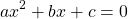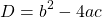# Chapter 6

## Chapter 6

### Exercises

1. Using the top-down method, please describe an algorithm for a program to solve the real roots of a quadratic equationYour algorithm should ask the user for the coefficients. To calculate the roots of the equation, your algorithm should calculate the discriminant D given by:If D > 0, the algorithm displays a message: “The equation has two roots” and then displays the roots.
If D = 0, the algorithm displays a message: “The equation has one root”, and then displays the root.
If D < 0, the algorithm displays a message: “The equation has no real roots”. Create a flowchart for your algorithm.
2. Using the top-down method, please describe an algorithm for a program that calculates the cost of a car rental according to the following price schedule:

The algorithm asks the user to enter the rental period and type of car. The algorithm should display the appropriate cost. If a period longer than 60 days is entered, a message “Rental is not available for more than 60 days” should be displayed. If a rental period of less than 6 days is entered for Class D, a message “Class D cars cannot be rented for less than 6 days” should be displayed. Create a flowchart for your algorithm.